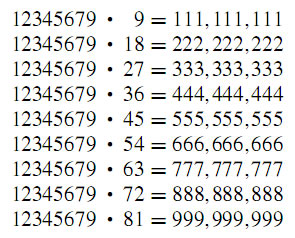### 美妙、有趣的數學(一) 數學的數字之美在貝殼村每天都能看到許多網友們用他們辛勤的勞動，呈現出一篇篇精彩的（遊記、散文、小說、科學、哲理、教育及幽默的短文等）文章，潛移默化地使我們的品性、修養和內涵潤物細無聲地積澱起來；一幅幅畫作和攝影作品，讓人賞心悅目；一首首詩歌，動人心弦；一曲曲悅耳動聽的音樂，激發撫慰我們的情懷。哲學使人獲得智慧，科學可改善物質生活。這些好的文學藝術作品，通過不同的表現手法來詮釋人們對美的理解，對美的嚮往，陶冶我們的情操，愉悅我們的心情。

那麼數學，在我們的眼中是什麼樣的呢？是枯燥無味的？困難的？畏懼的？

當然不是啦，數學與文學、繪畫、哲學、宗教、美學、音樂、人文科學、自然科學等文化領域都有著內在的聯繫。

數學有其多種美：統一美、諧調、對稱美、簡潔美、奇異美、應用美等。

讓我們先看看下面的數字之美，領略數學世界的美妙。

1x8+1=9

12x8+2=98

123x8+3=987

1234x8+4=9876

12345x8+5=98765

123456x8+6=987654

1234567x8+7=9876543

12345678x8+8=98765432

123456789x8+9=987654321

1x9+2=11

12x9+3=111

123x9+4=1111

1234x9+5=11111

12345x9+6=111111

123456x9+7=1111111

1234567x9+8=11111111

12345678x9+9=111111111

123456789x9+10=1111111111

9x9+7=88

98x9+6=888

987x9+5=8888

9876x9+4=88888

98765x9+3=888888

987654x9+2=8888888

9876543x9+1=88888888

98765432x9+0=888888888

1x1=1

11x11=121

111x111=12321

1111x1111=1234321

11111x11111=123454321

111111x111111=12345654321

1111111x1111111=1234567654321

11111111x11111111=123456787654321

111111111x111111111=12345678987654321

數學中的這些美正是激發我們的孩子們學習數學的源泉，用數學美來激起他們的學習興趣更是美不勝收，他們一旦感受到數學的美，就會帶著高漲的情緒學習和思考，能夠達到事半功倍的功效。

（我在Word上把每一個數字都 highlight了顏色的，看起來五顏六色的，更好看些，copy過來全部都是黑色了，也沒有時間一個一個再換顏色，只好將就這樣了）。

美妙、有趣的數學(二) 神奇的斐波那契與黃金分割：https://big5.backchina.com/blog/302989/article-233257.html

## 全部作者的其他最新日誌

### 發表評論 評論 (91 個評論)OMG! 蘭黛，你也太神奇了。到那裡搞來的。沒想到數學可以這麼漂亮。trunkzhao: 數字，幾何都很美妙。trunkzhao: 數字，幾何都很美妙。fanlaifuqu: 第一個有關三角的公式真沒看懂！jc0473: 震驚，數字真是太奇妙了tea2011: 太奇妙了，〜〜〜〜fanlaifuqu: 第一個有關三角的公式真沒看懂！jc0473: 謝謝蘭妹分享！我讀書時就是數學好，每次考試只用一半時間就交卷了，粗心小錯還是有點兒塗鴉板

Copyright © 2001-2013 海外華人中文門戶：倍可親 (http://big5.backchina.com) All Rights Reserved.